# Design and Analyze Band Stop Filter using `pcbComponent`

This example shows you how to design and analyze band stop filter using the `pcbComponent` object. Design the band stop filter with a fractional bandwidth (FBW) of 1.0 at a midband frequency ${f}_{0}$ of 2.5 GHz for the band-edge frequencies of ${f}_{1}$ = 1.25 GHz and ${f}_{2}$ = 3.75 GHz as defined in Figure 6.11b of reference .

#### Design Band Stop Filter

Design the microstrip band stop filter based on a three-pole (n = 3) Chebyshev lowpass prototype with 0.05 dB passband ripple. The element values of the lowpass prototype are

• ${g}_{0}$ = ${g}_{4}$ = 1.0

• ${g}_{1}$ = ${g}_{3}$ = 0.8794

• ${g}_{2}$ = 1.1132

Using the design equations Eq.(6.30) given in refrence  on page no. 182 for n = 3 and ${Z}_{0}$ = 50 Ω as , you can obtain

• ${Z}_{A}$ = ${Z}_{B}$ = 50 ohm

• ${Z}_{1}$ = ${Z}_{3}$ = 106.8544 ohm

• ${Z}_{12}$ = ${Z}_{23}$ = 93.9712 ohm

• ${Z}_{2}$ = 44.9169 ohm

Choose a commercial substrate `(RT/D 6006)` with a relative dielectric constant of 6.15 and thickness of 1.27 mm. Calculate the microstrip widths using the microstrip design equations given in Chapter 4 of reference . The figure shows the schematic diagram of the microstrip band stop filter  representing various feature dimensions.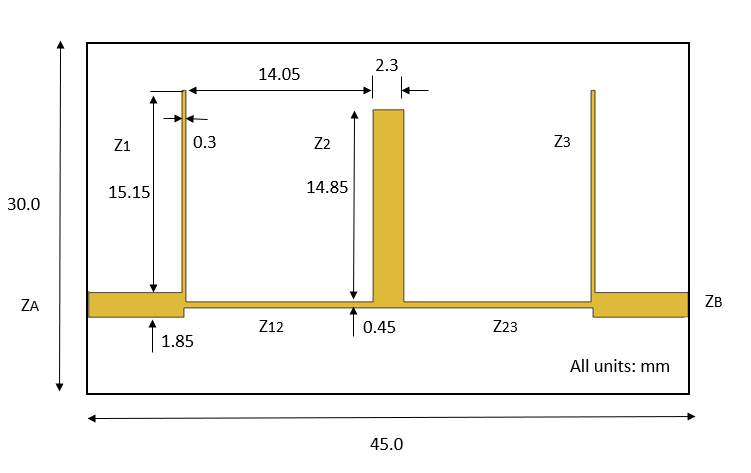Use the `traceRectangular` object to create `ZA`,` Z1`, `Z12.` Perform a Boolean add operation for the microstrip shapes `ZA`, `Z1`, `Z12 `and create a `LeftSection `object. Visualize the `LeftSection` using `show `function.

```ZA_Width = 1.85e-3; ZA_Length = 7e-3; Z1_Length = 0.3e-3; Z1_Width = 15.15e-3; Z12_Length = 14.05e-3; Z12_Width = 0.45e-3; Z2_Length = 2.3e-3; Z2_Width = 14.85e-3; gndL = 45e-3; gndW = 30e-3; ZA = traceRectangular(Length = ZA_Length+Z1_Length/2,Width = ZA_Width,... Center = [-gndL/2+ZA_Length/2+Z1_Length/4 0]); Z1 = traceRectangular(Length = Z1_Length,Width = Z1_Width+ZA_Width/2,... Center = [-gndL/2+ZA_Length+Z1_Length/2 (Z1_Width/2+ZA_Width/4)]); Z12 = traceRectangular(Length = Z12_Length+Z1_Length,Width = Z12_Width,... Center = [-gndL/2+ZA_Length+Z1_Length/2+Z12_Length/2 0]); LeftSection = ZA+Z1+Z12; figure; show(LeftSection);```Use the `copy`, `rotateZ` and `rotateX` functions on `LeftSection` object to create a `RightSection`. Visualize the `RightSection` object.

```RightSection = copy(LeftSection); RightSection = rotateZ(RightSection,180); RightSection = rotateX(RightSection,180); figure; show(RightSection);```Perform a Boolean add operation for the shapes `LeftSection `and `RightSection` to create a `combineSection `object. Use the `traceRectangular` object to create centerArm `Z2`. Perform a Boolean add operation for the shapes `combineSection`, `Z2`, and create a `filter `object. Visualize the `filter` object.

```combineSection = LeftSection + RightSection; Z2 = traceRectangular(Length = Z2_Length,Width = Z2_Width,... Center = [0 -Z12_Width/2+Z2_Width/2]); filter = combineSection + Z2; show(filter);```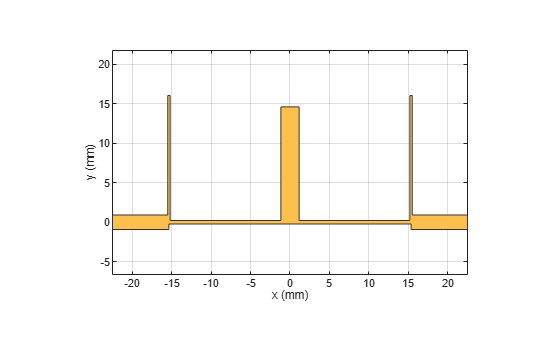Define the substrate parameters and create a dielectric to use in the `pcbComponent` of the designed filter. Create a groundplane using the `traceRectangular` shape.

```substrate = dielectric(EpsilonR = 6.15,LossTangent = 0.0027,... Name = "custom",Thickness = 1.27e-3); ground = traceRectangular(Length = gndL,Width = gndW,... Center = [0,6e-3]);```

#### Create PCB Filter Using `pcbComponent`

Use the `pcbComponent` to create a filter PCB. Assign the dielectric and ground plane to the `Layers` property of the `pcbComponent`. Assign the `FeedLocations` to the edge of the feed ports. Set the `BoardThickness` to 1.27 mm on the `pcbComponent` and visualize the filter.

```pcb = pcbComponent; pcb.BoardShape = ground; pcb.BoardThickness = 1.27e-3; pcb.Layers ={filter,substrate,ground}; pcb.FeedDiameter = ZA_Width/2; pcb.FeedLocations = [-gndL/2 0 1 3;gndL/2 0 1 3]; figure; show(pcb);```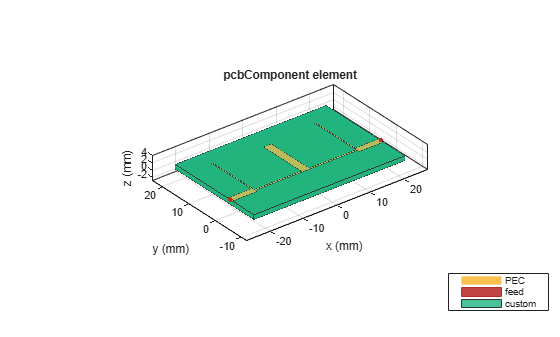#### Plot and Analyze the S-Parameters

Use the `sparameters` function to calculate the s-parameters for the band stop filter and plot it using the `rfplot` function.

```spar = sparameters(pcb,linspace(0.1e9,6e9,50)); figure; rfplot(spar);```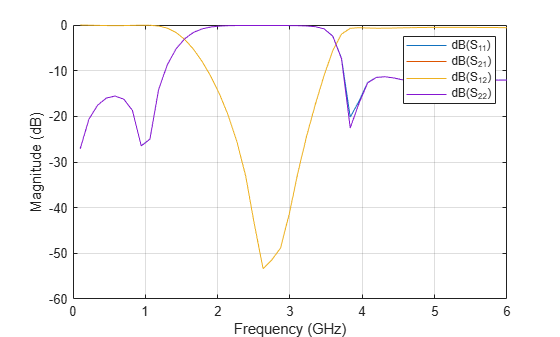As there are four curves in the result, let us analyze the results.

Analyze the values of ${S}_{12}$, and ${S}_{11}$ to understand the behavior of band stop filter.

```figure rfplot(spar,1,1:2)```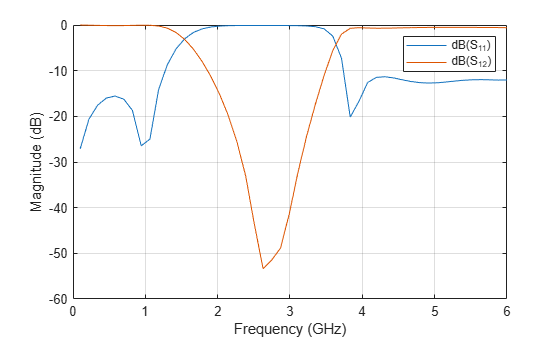The result shows that the filter has center frequency ${f}_{0}$ = 2.5 GHz for the band-edge frequencies ${f}_{1}$ = 1.75 GHz and ${f}_{2}$ = 3.4 GHz. The ${S}_{11}$ values are close to 0 dB and ${S}_{12}$ values are less than -10 dB between frequencies ${f}_{1}$ = 1.4 GHz and ${f}_{2}$ = 3.4 GHz. The designed filter therefore has stopband response. The shift in band-edge frequencies ${f}_{1}$ and ${f}_{2}$ might be due to use of different full wave numerical solver used for EM simulation.

#### Visualize Charge and Current Distribution

Use the `charge` function to visualize the charge distribution on the metal surface and dielectric of band stop filter.

```figure; charge(pcb,2.4e9);``````figure; charge(pcb,2.4e9,'dielectric');```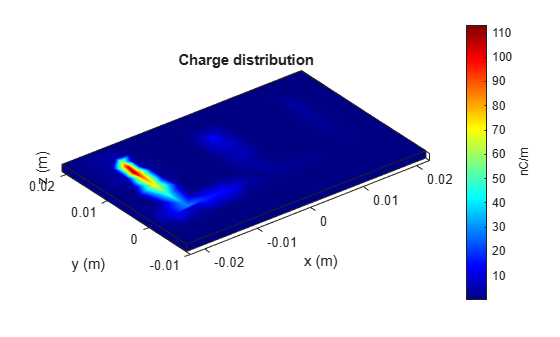Use the `current` function to visualize the current distribution on the metal surface and the volume polarization currents on dielectric of band stop filter

```figure; current(pcb,2.4e9);``````figure; current(pcb,2.4e9,'dielectric');```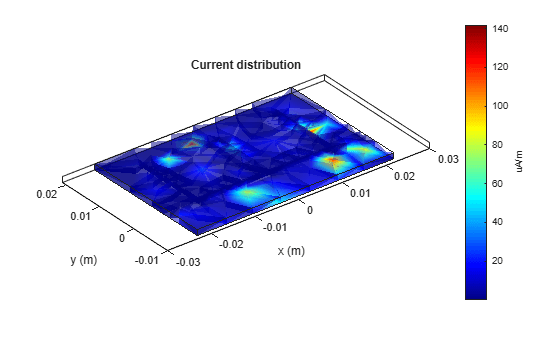### References

 Jia-Sheng Hong "Microstrip Filters for RF/Microwave Applications", p. 184, John Wiley & Sons, 2nd Edition, 2011.